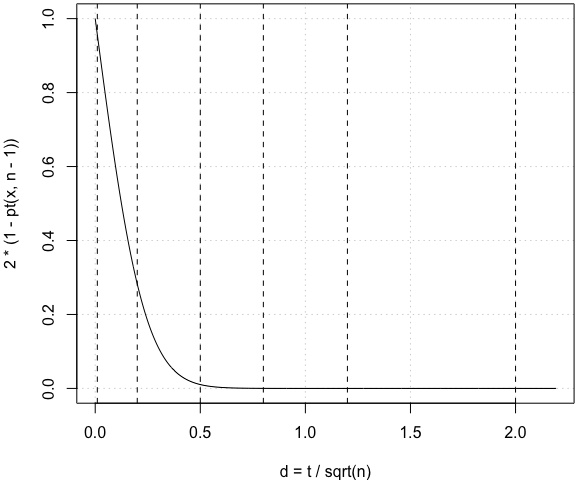# Cohen’s d

### 谢益辉 2018-02-12

1. 这概念压根儿没什么卵用；

2. 现今的统计博士生研究的东西太专了；

``````n = 30  # 样本量（只是一个例子）
x = seq(0, 12, 0.01)
par(mar = c(4, 4, 0.2, 0.1))
plot(x/sqrt(n), 2 * (1 - pt(x, n - 1)), xlab = "d = t / sqrt(n)", type = "l", panel.first = grid())
abline(v = c(0.01, 0.2, 0.5, 0.8, 1.2, 2), lty = 2)
``````